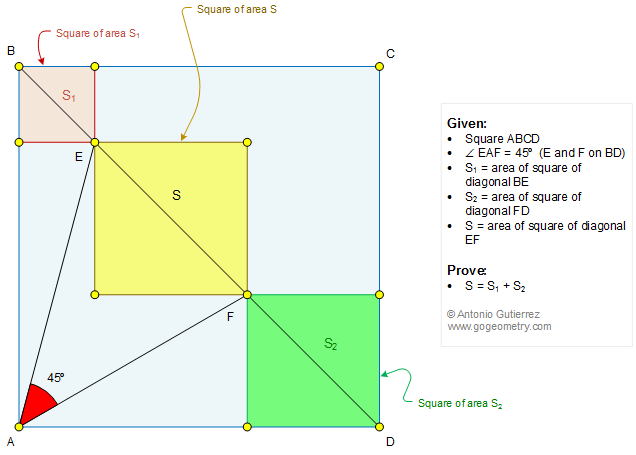# Geometry Problem 1338: Four Squares, Diagonals, Angle, 45 Degrees, Areas. Math Infographic.

In the figure bellow, ABCD is a square and the measure of the angle EAF is 45 degrees (E and F lie on BD). If S1, S2, and S are the areas of the squares of diagonals BE, FD, and EF, respectively, prove that S = S1 + S2.